Latest Banking jobs   »   Quantitative Aptitude Quiz For Bank Mains...

# Quantitative Aptitude Quiz For Bank Mains Exams 2021- 20th February

Directions (1- 2): The following questions are accompanied by two statements (I) and (II). You have to determine which statements(s) is/are sufficient/necessary to answer the questions.
(a) Statement (I) alone is sufficient to answer the question but statement (II) alone is not sufficient to answer the question.
(b) Statement (II) alone is sufficient to answer the question but statement (I) alone is not sufficient to answer the question.
(c) Both the statements taken together are necessary to answer the question, but neither of the statements alone is sufficient to answer the question.
(d) Either statement (I) or statement (II) by itself is sufficient to answer the question.
(e) Statements (I) and (II) taken together are not sufficient to answer the question.

Q1. A & B are two workers. If both A & B start work alternatively starting with A, then in how many hours work will be completed?
I. Efficiency of B is 50% more than A. If both A & B work together they can complete the task in 12 hours.
II. Ratio of efficiency of A : B is 2 : 3 and C is 25% more efficient than A. If C work for first hour and second hour all three work together and this process continued till the end, then work will be completed in 12 hours.

Q2. Total number of boys in school is how much more than total number of girls in school in 2016?
I. Total number of students in 2015 is 1152 and number of boys & girls increased by 20% and 25% in the year 2016 compared to year 2015.
II. If 50% of total number of boys and 75% of total number of girls appeared in the 2015 exam, then total number of girls appeared in the exam is 64 more than that of total number of boys appeared in the 2015 exam.

Directions (3 -7): The following questions are accompanied by two statements (I) and (II). You have to determine which statements(s) is/are sufficient/necessary to answer the questions.
(a) Statement (I) alone is sufficient to answer the question but statement (II) alone is not sufficient to answer the questions.
(b) Statement (II) alone is sufficient to answer the question but statement (I) alone is not sufficient to answer the question.
(c) Both the statements taken together are necessary to answer the questions, but neither of the statements alone is sufficient to answer the question.
(d) Either statement (I) or statement (II) is sufficient to answer the question.
(e) Statements (I) and (II) together are not sufficient to answer the question.

Q3. What is annual salary of Anurag?
I. If Anurag deposited 45% of his monthly salary at the rate of 20% half yearly for one year at compound interest, then he will get an amount which is Rs. 88 less than his remaining monthly salary.
II. If Anurag divided 55% of his annual salary between his son & daughter in the ratio of 13 : 11, then difference between remaining annual salary of Anurag and share of his son will Rs. 29200.

Q4. If longer train added three more compartment of 15 m each, then find in what time both trains cross each other running in opposite direction
I. Ratio between length of two trains is 4 : 5 and speed of longer trains & shorter train is 81 km/hr & 108 km/hr respectively.
II. Longer & shorter trains cross each other in 36 sec running in same direction.

Q5. A seller marked up price of a table 60% above cost price and give three discounts of 12.5%, 20% and d% respectively. Find ‘d’.
I. Seller make a profit 7.52%
II. Cost price of table is Rs. 1200 and it sold in Rs. 1290.24 after allowing three given discounts.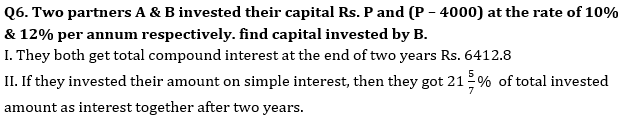Q7. In a River there are two boats P and Q, where boat P covers 30 km downstream and boat Q covers 30 km upstream. find the speed of boat Q in still water.
I. Boat Q takes 2 hours more than boat P in covering the given distance.
II. Sum of speed of boat P in still water and boat Q in still water is 16 km/hr and speed of water current is 1 km/hr.

Directions (8-10): In the given questions, two quantities are given, one as ‘Quantity I’ and another as ‘Quantity II’. You have to determine relationship between two quantities and choose the appropriate option:

Q8. Radius of cone is 4 cm less than side of square, which area is 324 cm2. Height of cone is 5 cm more than radius of circle, which circumference is 44 cm.
Quantity I – Volume of cone.
Quantity II – 2488 cm3
(a) Quantity I > Quantity II
(b) Quantity I < Quantity II
(c) Quantity I ≥ Quantity II
(d) Quantity I ≤ Quantity II
(e) Quantity I = Quantity II or no relation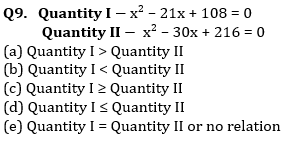Q10. 240 meters & 210 meters long train running in opposite direction cross each other in 6 sec. Ratio between speed of longer train and smaller train is 7 : 8 and faster train cross a platform in 9 sec.
Quantity I – Time taken (in sec) by slower train to cross a bridge, which is 60 meters longer than platform.
Quantity II – 16 sec
(a) Quantity I > Quantity II
(b) Quantity I < Quantity II
(c) Quantity I ≥ Quantity II
(d) Quantity I ≤ Quantity II
(e) Quantity I = Quantity II or no relation

There are three sitting halls, three pantry, three meeting rooms and one HR & one CEO room in the office of Adda247. All halls, pantry, meeting rooms, HR room & CEO rooms are rectangular in shape and area of each hall is same, each pantry is same, each meeting room is same and area is different for HR & CEO room. Breadth of hall & pantry are 33 m & 15 m respectively, while ratio of length of hall to that of pantry is 22 : 7. The difference between perimeter of a hall & a pantry is 126 m, while area of a meeting room having breadth of 14 m is 77 m2 less than that of area of a pantry room. Ratio of magnitude of perimeter of HR room to area of that room is 19 : 88 and breadth of that room is 16 m. Length & breadth of CEO room is 4 m & 2 m more than that of length and breadth of HR room respectively. (Consider only 2D figure)

Q11. The radius of a cylindrical vessel is equal to half of the length of a pantry. Its volume is 4158 m3 and cost of polishing it is Rs. 5 per m2, then find the total cost of polishing the vessel including its top and bottom surfaces.
(a) 7225 Rs.
(b) 7425 Rs.
(c) 7050 Rs.
(d) 7825 Rs.
(e) 7625 Rs.

Q12. The ratio of radius of two circular parks is 5: 6 and sum of circumference of both circular parks is 154m more than perimeter of a CEO room. If cost of fencing per meter with wire is Rs. 25, then find the total cost of fencing the smaller circular park?
(a) 2560 Rs.
(b) 2456 Rs.
(c) 2226 Rs.
(d) 2288 Rs.
(e) 2750 Rs.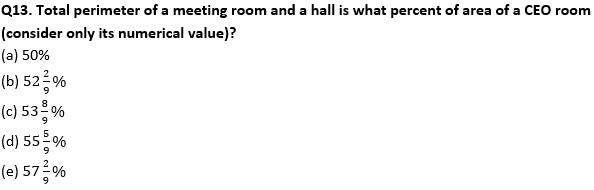Q14. All three meeting rooms are rebuilt so that breadth of each meeting room is increased by 100% and length is decreased by 10 m. If each meeting room is divided into four square cabins and decorated with wood which costs Rs 12.5 per/m2, then find the total cost of decoration of all square cabins built in the three meeting rooms?
(a) 7290 Rs.
(b) 7230 Rs.
(c) 7240 Rs.
(d) 7350 Rs.
(e) 7560 Rs.

Q15. Find the difference between perimeter of a HR room & perimeter of a pantry?
(a) 4 m
(b) 8 m
(c) 12 m
(d) 16 m
(e) 18 m

Practice More Questions of Quantitative Aptitude for Competitive Exams:

###### Bank Mains Cracker Study Plan 2021 : Check Now

Solutions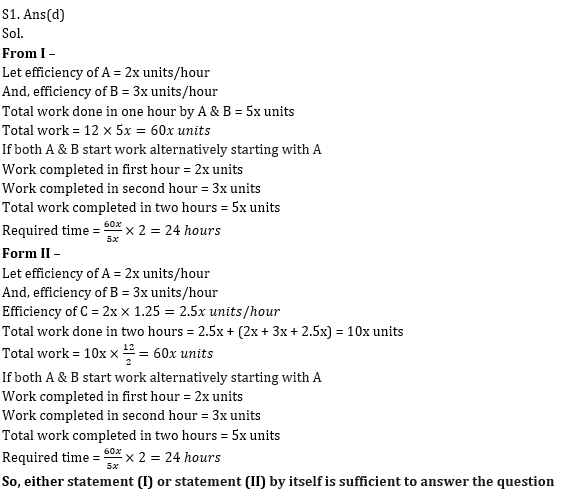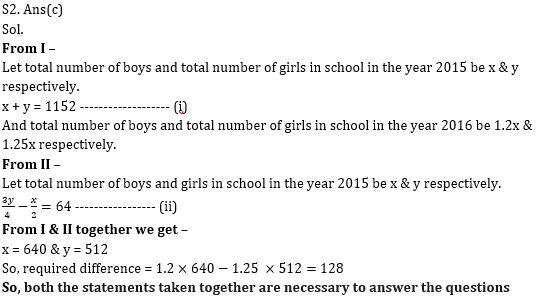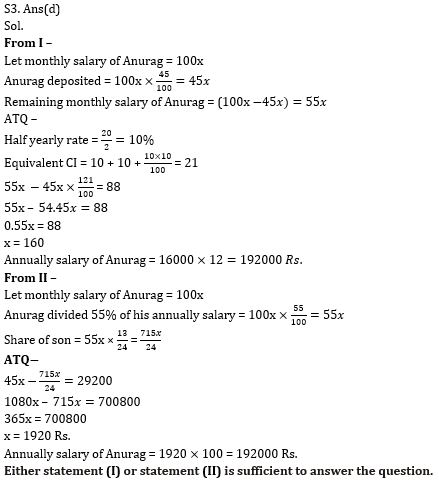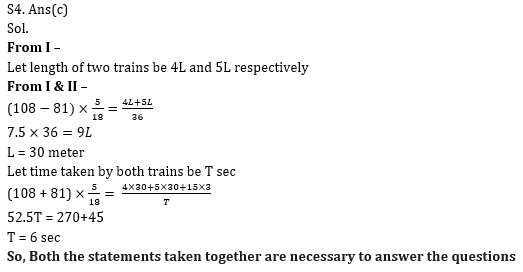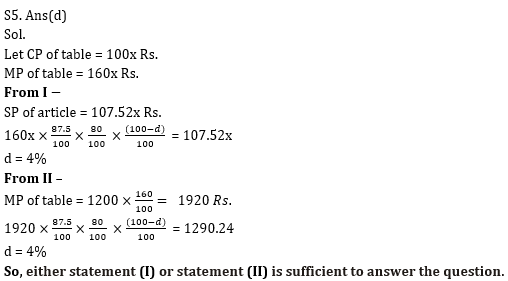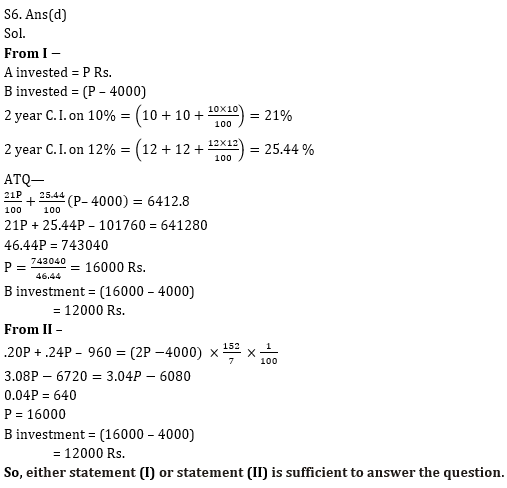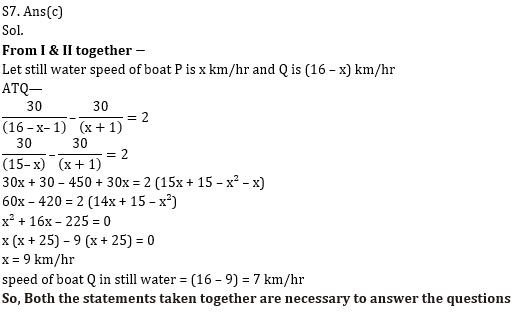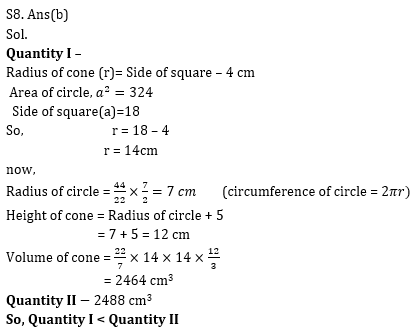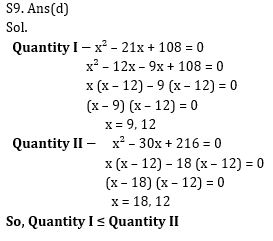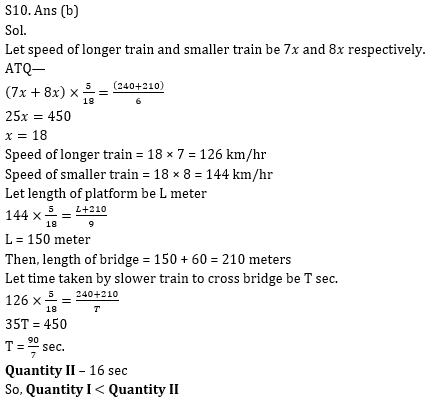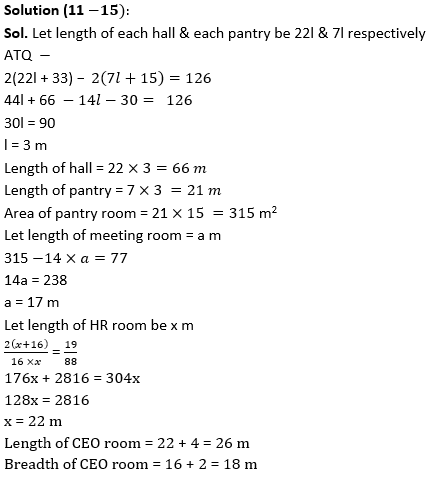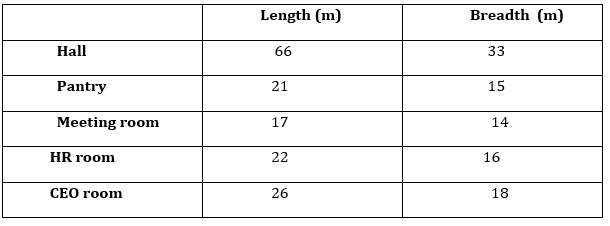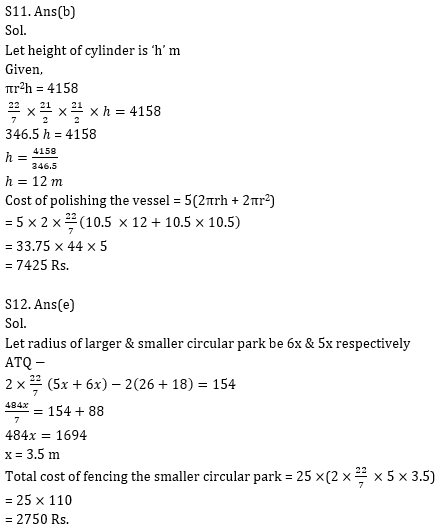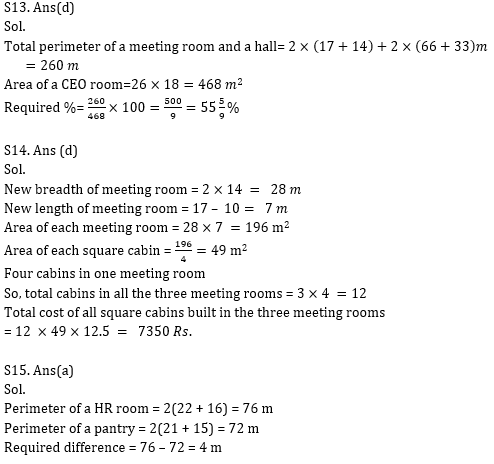Practice with Online Test Series for Bank Mains 2021:×

Thank You, Your details have been submitted we will get back to you.Join India's largest learning destination

What You Will get ?

•Job Alerts
•Daily Quizzes
•Subject-Wise Quizzes
•Current Affairs
•Previous year question papers
•Doubt Solving session

ORJoin India's largest learning destination

What You Will get ?

•Job Alerts
•Daily Quizzes
•Subject-Wise Quizzes
•Current Affairs
•Previous year question papers
•Doubt Solving session

ORJoin India's largest learning destination

What You Will get ?

•Job Alerts
•Daily Quizzes
•Subject-Wise Quizzes
•Current Affairs
•Previous year question papers
•Doubt Solving session

Enter the email address associated with your account, and we'll email you an OTP to verify it's you.Join India's largest learning destination

What You Will get ?

•Job Alerts
•Daily Quizzes
•Subject-Wise Quizzes
•Current Affairs
•Previous year question papers
•Doubt Solving session

Enter OTP

Please enter the OTP sent to
/6

Did not recive OTP?

Resend in 60sJoin India's largest learning destination

What You Will get ?

•Job Alerts
•Daily Quizzes
•Subject-Wise Quizzes
•Current Affairs
•Previous year question papers
•Doubt Solving sessionJoin India's largest learning destination

What You Will get ?

•Job Alerts
•Daily Quizzes
•Subject-Wise Quizzes
•Current Affairs
•Previous year question papers
•Doubt Solving session

Almost there

+91Join India's largest learning destination

What You Will get ?

•Job Alerts
•Daily Quizzes
•Subject-Wise Quizzes
•Current Affairs
•Previous year question papers
•Doubt Solving session

Enter OTP

Please enter the OTP sent to Edit Number

Did not recive OTP?

Resend 60

By skipping this step you will not recieve any free content avalaible on adda247, also you will miss onto notification and job alerts

Are you sure you want to skip this step?

By skipping this step you will not recieve any free content avalaible on adda247, also you will miss onto notification and job alerts

Are you sure you want to skip this step?